# Written methods of Division

Children sometimes struggle with the concept of division. I begin by relating it to sharing, mainly food, pizzas or chocolate cake, with their friends and draw lots of pictures to help them visualise what is happening.
How many pieces will you have to cut the cake into to get an equal share?
Four friends plus you means that you have to cut the cake into 5 or divide it by 5.

If the cake was cut into 10 equal pieces, how may pieces would each person get? 10 ÷ 5 = 2.

If the cake was cut into 11 pieces, how many pieces would each person get and what would
be left over or remaining? 10 ÷ 5 = 2 remainder 1.

But what does the remainder mean? To share the cake properly, you would have to share the remaining piece as well - which is 1 ÷ 5 or 1/5 - a fifth. So each child would get 2 whole pieces and one fifth of a piece - 2 1/5 slices.

Timestables are again very important here, as is the idea of "inverse" or opposite. If you multiply an amount by a certain number, say 5 x 3 = 15, and then divide the answer by the same number, 15 ÷ 3, you get 5, back where you started.

So a secure knowledge of timestables is essential to be able to divide. Take 30 ÷ 6 as an example. By recalling the 6 times table, you know that 5 x 6 = 30, so your answer is 5.

### Remember "Times and Divide" are Opposites (Inverse).

Try these worksheets on division facts from Mathsblog to practise.
Know Division Facts 1 and Know Division Facts 2

Division can also be thought of as repeated subtraction. 20 ÷ 5 is the same as
20 - 5 =15      15 - 5 = 10      10 -5 =5      5 - 5 = 0
5 was subtracted 4 times - so 20 ÷ 5 = 4, with nothing remaining. 20 ÷ 5 = 4

22 ÷ 5 is  ...  22 - 5 = 17      17 - 5 = 12     12 - 5 = 7     7 - 5 = 2
5 was subtracted 4 times - so 20 ÷ 5 = 4, this time with 2 remaining.
22 ÷ 5 = 4 r 2 (remainder 2 means 2/5) so 22 ÷ 5 = 4 2/5.

# The Chunking Method of Division

This method takes the repeated subtraction idea a step further and is the way that written methods of subtraction are introduced at KS2.

I explain it by working through a problem - say your teacher has given you £4.00 and asked you to buy as many pencils as you can from the shop and bring back the change.
Pencils cost 30p. First question is what is £4 in p. ... 100p is £1 - so £4 = 4 x 100 = 400p.

So how many 30p pencils can you buy with 400p?

What facts do you know about 30? ... 30 x 10 =300 - so if you bought 10 pencils it would cost you 300p (£3). How much change would you get? ... 400 - 300 = 100 (£1)

Can you buy some more pencils with the change? What facts do you know about 30?
3 x 30 = 90 ... you can buy another 3 pencils with the 100p (£1) and have 10p left over.

How many pencils have you bought? ... 10 + 3 = 13 with 10p remaining as change.

Lets write that down.

Write out the calculation - 400 ÷ 30

What facts do you know about 30?
10 x 30 =300
400 - 300 =100 remaining.

Does 30 go into 100? ... Yes

What facts do you know about 30?
3 x 30 = 90
100 - 90 =10 remaining.

Does 30 go into 100? ... No

Add the number of 30's you have used ... 10 +3 = 13

Step 1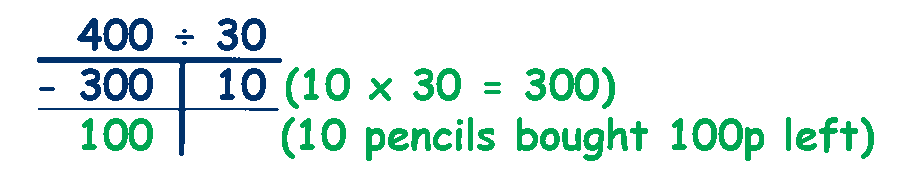Step 2Step 3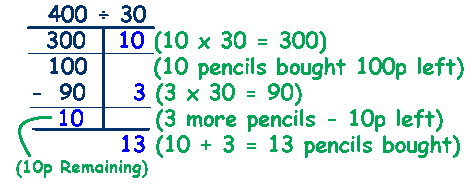# The Long Division Method

Children move on to the Long Division method. It is the preferred method when numbers get more complex with 2 or more digits. This is sometimes called the 'Bus Stop' method.

Write out the calculation - 856 ÷ 4

Long division works by dealing with the largest column 1st - in this case the 8 (800).

How many times does 4 go into 8? ... from the 4 times table ..2 x 4 = 8. Write the 2 above the 8 and the result, 8, is written below the 8.

Work out the remainder by subtracting 8 from 8, 8-8=0 Now bring the 5 down to make 05 the next number to divide into.

How many times does 4 go into 5? ... from the 4 times table ..1 x 4 = 4. Write the 1 above the 5 and the result, 4, is written below the 5.

Work out the remainder by subtracting 4 from 5, 5-4=1 Now bring the 6 down to make 16 the next number to divide into.

How many times does 4 go into 16? ... from the 4 times table ..4 x 4 = 16. Write the 4 above the 6 and the result, 16, is written below the 16.

Finally, subtract 16 from 16, 16-16=0, which means that there is no remainder.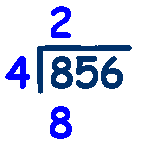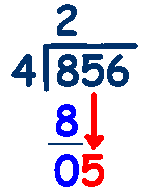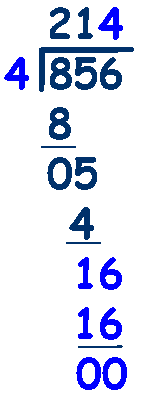TU ÷ U division worksheet from Mathsblog.

HTU ÷ TU multiplication
worksheet
from Mathsblog.